The last article on the topics of “Game Architecture for Card Game” series will focus on the amazing “Race for the Galaxy” AI. Even though Keldon Jones released his RFTG AI source code back in 2009 [Jones09], it was using neural networks and reinforcement learning to train the game AI, way before DeepMind’s Alpha Go success that drew the world’s attention to reinforcement learning.

At the heart of the card game architecture is the AI that keeps the human player engaged and provides the endless replayability of a game. We must provide the AI agent (either a single or a group of players) the ability to make intelligent decisions according to the game rules and logic. The AI action will be the movement that affects the future game states. The reward of the decision is simple, “winning” the target game.Figure. Game Architecture Overview - The components are grouped according to their functional roles in the system. The functional roles are (1) Game Story and Game Asset, (2) Game Model, (3) Game Engine, (4) Game Interface, (5) Game AI, (6) Game Physics (only for physics based game), and (7) Hardware Abstraction. When studying any game source code, this architecture will help to classify their functional roles

To train a Reinforcement Learning (RL) agent how to play a card game intelligently, a full-fledged game environment has been put in place and needs to capture all the mechanics and rules so that the agent can interact with the game like a real human player would do. The hardest part is to develop the game that can play intelligently against itself. With that thought, researches using RL in card games are gaining a lot of popularity, such as the card games of Easy21 [Amrouche20], UNO [Pfann21]. The RLCard Toolkit [Daochen19], goes even one step further by providing a general RL framework for researching card games. In this article, we shall continue focus on “Race for the Galaxy” card game.

However, the RFTG card game’s rule complexity is difficult to express in a well-defined game state representation, unlike Easy21 or UNO simplicity. We must raise the level of abstraction in order to describe RFTG AI. We are hoping to guide readers in the right direction with the following outline.

## RFTG Python Development

Interest reader can find the full development set up instruction, Python source code and Jupyter notebook experiments described in this article from [Cheung21].

### Jupyter Notebook Experiments (Part 3)

The development experiments on (Part 3) are recorded in the Jupyter Notebook rftg_ai.ipynb to quickly run the code samples. Inside Visual Studio code, install the Microsoft’s “Jupyter” extension. When activate the rftg_ai.ipynb inside VScode, change the Python kernel to use rftg that has been setup from the code README.md instructions.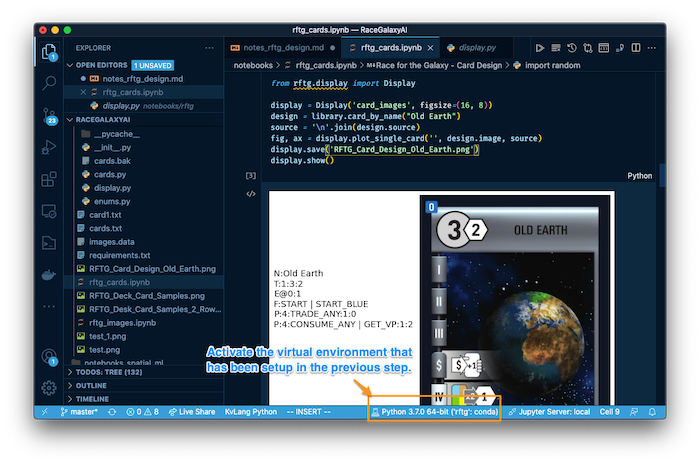### Installation for AI Notebook

The following Python modules are required to run this AI notebook rftg_ai.ipynb.

pip install keras==2.4.3
pip install tensorflow==2.5.0
pip install pydot


In addition, we need to define the .keras/keras.json to use the tensorflow backend.

 {
"image_data_format": "channels_last",
"epsilon": 1e-07,
"floatx": "float32",
"backend": "tensorflow"
}


## Temporal Difference - Reinforcement Learning

Collecting the information provide by both Keldon’s post and Temple Gate’s blog post, we learned that RFTG AI follows Tesauro’s TD-Gammon ideas using Temporal Difference (TD) neural network. And yes, neural network and reinforcement learning techniques are applied to games since the ’90s.

One of the biggest attraction of reinforcement learning, it does not require a pre-defined model (aka. model-free) and no human input needed to generate the training data. The neural network learns by repeatedly playing with itself, for instance, RFTG AI was trained iteratively over 30,000 simulated games to find the weights for neural network nodes. The innovative idea of this learning algorithm consists of updating the weights in its neural net after each turn to reduce the difference between its evaluation of previous turns’ board positions, its evaluation of the present turn’s board position—hence “temporal-difference learning”.

During network training, AI examines on each turn a set of possible legal moves and all their possible responses (via the optimal policy), feeds each resulting game state into its evaluation function, and chooses the action that leads to the player got the highest score. TD’s innovation was in how it learned its evaluation function incrementally using reinforcement learning.

After each turn, the learning algorithm updates each weight in the neural net according to the following rule:

$w_{t+1} - w_t = \alpha(Y_{t+1} - Y_t)\sum_{k=1}^{t}\lambda^{t-k} \nabla_w Y_k$

where:

• $w_{t+1} - w_t$ is the amount to change the weight from its value on the previous turn.
• $Y_{t+1} - Y_t$ is the difference between the current and previous turn’s board evaluations.
• $\alpha$ is a “learning rate” parameter.
• $\lambda$ is a parameter that affects how much the present difference in board evaluations should feed back to previous estimates. $\lambda = 0$ makes the program correct only the previous turn’s estimate; $\lambda = 1$ makes the program attempt to correct the estimates on all previous turns; and values of $\lambda$ between 0 and 1 specify different rates at which the importance of older estimates should “decay” with time.
• $\nabla_w Y_k$ is the gradient of neural-network output with respect to weights: that is, how much changing the weight affects the output.

For more reinforcement learning, reader can consult the excellent tutorial by Tambet Matiisen [Matiisen15], DeepMind’s RL lectures by David Silver [Silver17] and the classic RL textbook by Sutton [Sutton17].

### Game State

The game state can represent any information available to the decision-maker, that is useful to describe the current situation of the game. This could be the type of cards a player holds, the number of cards the opponent holds, or information regarding cards that have already been played. Without a doubt, RFTG is a complex card game, due to its elaborate set of rules and phases of action.

By reading the list of input node names, we can understand the game states are fed into the network. The game state can be,

• Game over
• VP Pool size
• Max active cards
• Type of cards at hand
• The number of cards at hand
• Player number of goods
• Opponent number of goods
• etc.

The list can go from 700s for a game of 2 players up to 1,800s for a game of 5 players (using expansion=6).

### Neural Networks

These inputs don’t just feed into one neural network. According to Keldon’s code, he got twelve unique models of neural networks each trained for a different set of expansions and player count. If we’re running a two-player game, the AI is on a different network than a three-player game. For each game model, there are two flavours of neural networks at work, each with its main function.

For example,

• Eval Network - file for eval weights for expansion 0 (base game) 2 (players) rftg.eval.0.2.net
• Role Network - file for role weights for expansion 0 (base game) 2 (players) rftg.role.0.2.net

Both network weights file format are the same; the contents are varying with,

• Input size, hidden layer size, output layer size
• For example rftg.eval.0.2.net : (704, 50, 2)
• For example rftg.role.0.2.net : (605, 50, 7)
• Number of training iterations
• The list of input node names
• The weights for each layer nodes

The following Python class Network is the network weights loader implementation.

import numpy as np

class Network:

def __init__(self):
pass

def network_name(self, network, expansion, players, advanced=False):
network_name = "rftg.%s.%d.%d%s.net" %(network, expansion, players, ("a" if advanced else ""))
return network_name

self.network = network
self.expansion = expansion
self.players = players
self.network_name = self.network_name(network, expansion, players, advanced)

filename = 'network/{}'.format(self.network_name)
fp = open(filename)
(input, hidden, output) = fp.readline().strip().split(' ')
print(input, hidden, output)
# read number of training iterations

self.num_input = int(input)
self.num_hidden = int(hidden)
self.num_output = int(output)

self.input_names = []
for i in range(0, self.num_input):
self.input_names.append(name)

self.input = np.zeros(shape=(self.num_input, 1))
print('Input Layer: ', self.input.shape)

self.hidden = np.ndarray(shape=(self.num_input, self.num_hidden))
for r in range(0, self.num_hidden):
for c in range(0, self.num_input):
print('Hidden Layer: ', self.hidden.shape)

self.output = np.ndarray(shape=(self.num_hidden, self.num_output))
for r in range(0, self.num_output):
for c in range(0, self.num_hidden):
print('Output Layer: ', self.output.shape)

# all done
fp.close()


For example, reading role network of expansion 0 with 2 players, not advanced game. The network file is rftg.role.0.2.net.

network = Network()


The network file information are printed.

605 50 7
Input Layer:  (605, 1)
Hidden Layer:  (605, 50)
Output Layer:  (50, 7)


#### Eval Network

The eval network output can be thought of each player’s chances to win. Each row corresponds to computing the eval network from the point of view of that player because certain things are known only to a given player, such as cards in hand. For example, the following eval network is for 2 players, i.e. the output layer has 2 output for each player winning scores.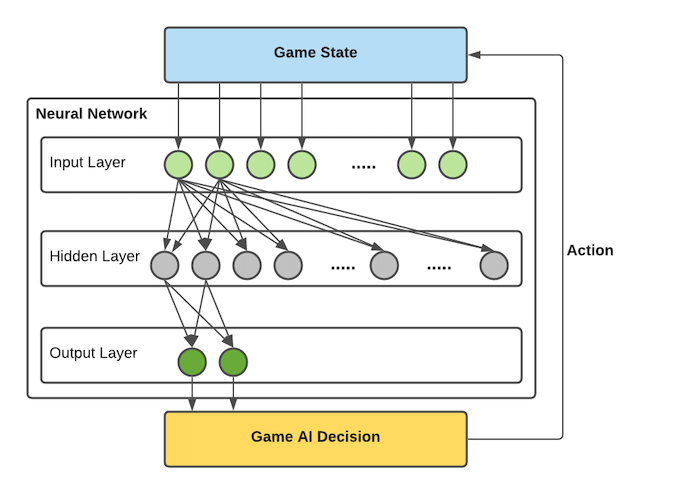Figure. Showing the fully connected eval network layers. (note: not all connections are drawn for less lines). The input layer nodes are the designed game states and the output layer nodes are the winning scores for each player (in this example, 2 nodes for 2 players).

#### Role Network

There is another important decision is choosing which action at the beginning of each turn. This is handled a bit differently. First, the AI predicts what each opponent is likely to do. This is done with a second role network. This network is very similar to the eval network, except that it has an output for each possible 7 types of action choice a player may make.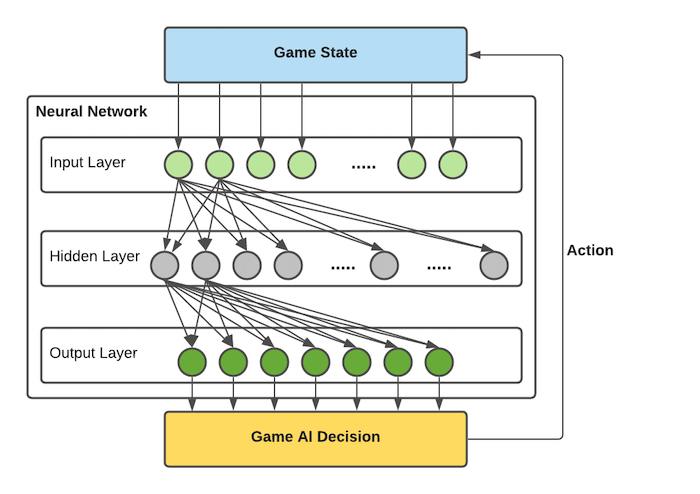Figure. Showing the fully connected role network layers. (note: not all connections are drawn for less lines). The input layer nodes are the designed game states and the output layer nodes are the 7 types of action choice.

### Decisions

Using the network is simple, gathering all the required game state as input to the network. Once the game state is scored by both networks, the scores represent the chance of player winning and the role of action choice, are handled by AI to make a decision for a action.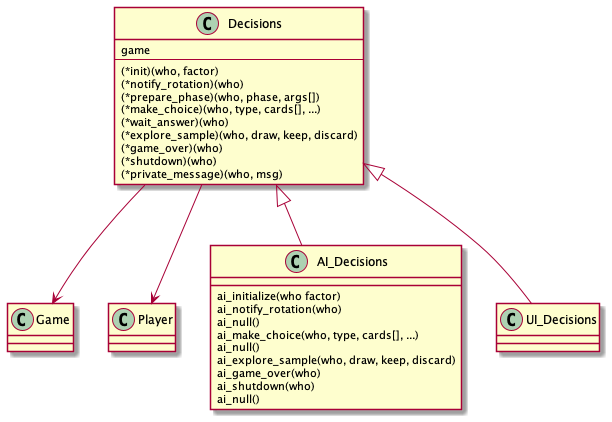Figure. the abstract superclass Decisions functions are overridden by the implementation of AIDecisions functions

As we have examined the Decision is an abstract superclass that defines all the decision operations that are implemented as UIDecision for human actors and are implemented by AIDecision for a computer AI actor. The cards, that the AI agent decides to play, represent the actions it is taking.

There are more logic to make a choice that we shall not drill into the details. The AI make_choice function choice_actions will map the Choice enum to the associative action function.

  def make_choice(self, who, type, **kwargs):
print('{} make_choice'.format(self.__class__.__name__))
choice_action = {
Choice.ACTION:  self.choose_action,
Choice.START:   self.choose_start,
Choice.PLACE:   self.choose_place,
Choice.PAYMENT: self.choose_payment,
Choice.SETTLE:  self.choose_settle,
Choice.CONSUME: self.choose_consume,
Choice.CONSUME_HAND: self.choose_consume_hand,
Choice.GOOD:    self.choose_good,
Choice.LUCKY:   self.choose_lucky,
Choice.WINDFALL: self.choose_windfall,
Choice.PRODUCE: self.choose_produce,
}


## Deep Neural Network using Keras

In this section, we proceed to use Keras: the Python Deep Learning API to rewrite the RFTG’s Neural Network. Although it felt overkill, the purpose is to illustrate using this powerful toolkit to achive greater network flexibility; Subsequently, we can potentially extend the network abilities, e.g. using LSTM (long short term memory) network. To learn Keras, we recommend the book by Keras designer, Francois Chollet [Chollet18].

### Define Keras Layers

Keras layer that will only accept as input 2D tensors where the first dimension is the network input size, e.g. 605 (axis 0, the batch dimension, is unspecified, and thus any value would be accepted). This layer will return a tensor where the first dimension has been transformed to be 50. Thus this layer can only be connected to a downstream layer that expects 50-dimensional vectors as its input.

The second layer didn’t receive an input shape argument—instead, it automatically inferred its input shape as being the output shape of the layer that came before.

The last layer uses a softmax activation. It means the network will output a probability distribution over the 7 different output classes—for every input sample, the network will produce a 7-dimensional output vector, where output[i] is the probability that the sample belongs to class i (i.e. role choice). The 7 scores will sum to 1.

from keras import models
from keras import layers

model = models.Sequential(name=network.network_name)
# input layer implicit in the input_shape data
# hidden layer
# output layer


### Visualize Model

The summary can be created by calling the summary() function on the model that returns a string that in turn can be printed.

model.summary()

Model: "rftg.role.0.2.net"
_________________________________________________________________
Layer (type)                 Output Shape              Param #
=================================================================
hidden (Dense)               (None, 50)                30300
_________________________________________________________________
output (Dense)               (None, 7)                 357
=================================================================
Total params: 30,657
Trainable params: 30,657
Non-trainable params: 0
_________________________________________________________________


The summary is useful for simple models, but can be confusing for models that have multiple inputs or outputs. Keras also provides a function to create a plot of the network neural network graph that can make more complex models easier to understand.

The plot_model() function in Keras will create a plot of your network.

from keras.utils.vis_utils import plot_model

plot_filename='{}.png'.format(network.network_name)
plot_model(model, to_file=plot_filename, show_shapes=True, show_layer_names=True)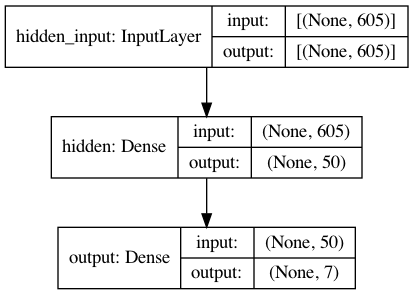We have load the RFTG role network weights. After defining the Keras model, we can assign the weights into the corresponding model’s layers

# find the DNN layer by name
hidden_layer = model.get_layer('hidden')
output_layer = model.get_layer('output')

# set the loaded network weights into the DNN layers
hidden_layer.set_weights([network.hidden, np.zeros(shape=hidden_layer.output_shape)])
output_layer.set_weights([network.output, np.zeros(shape=output_layer.output_shape)])


We can inspect the weights from the hidden layer.

hidden_layer.get_weights()

[array([[ 0.02684849, -0.07043163,  0.17830057, ...,  0.08084569,
-0.09834311,  0.3189879 ],
[ 0.03219581,  0.06759464, -0.08408735, ...,  0.08180485,
0.12012478,  0.03991435],
[-0.07090639, -0.01249493,  0.05813789, ...,  0.04127461,
0.0998257 , -0.01798295],
...,
[-0.8491952 , -0.30811298, -0.12133851, ...,  0.01339607,
-0.10589286,  0.00837689],
[ 1.2423377 , -0.0644151 ,  0.24686392, ...,  0.06034593,
0.04830525,  0.00200542],
[-0.5312858 ,  0.17594491,  0.3015494 , ..., -0.10620899,
-0.03211459, -0.07428143]], dtype=float32),
array([0., 0., 0., 0., 0., 0., 0., 0., 0., 0., 0., 0., 0., 0., 0., 0., 0.,
0., 0., 0., 0., 0., 0., 0., 0., 0., 0., 0., 0., 0., 0., 0., 0., 0.,
0., 0., 0., 0., 0., 0., 0., 0., 0., 0., 0., 0., 0., 0., 0., 0.],
dtype=float32)]


### Using Neural Network for Predictions (Scores)

After the network weights are loaded into Keras model, we can verify with the predict() method of the model instance returns a probability distribution over all 7 topics.

Let’s generate a random set of game state as the test input data.

random_input = np.random.randint(2, size=hidden_layer.input_shape)
x_input = np.array([random_input])


Our input vector will be 605-dimensions of (0 or 1).

array([[0, 1, 1, 0, 1, 0, 1, 0, 1, 1, 1, 1, 0, 0, 1, 0, 1, 1, 1, 1, 1, 0,
0, 0, 1, 0, 1, 1, 1, 0, 0, 1, 0, 1, 0, 1, 0, 0, 1, 1, 0, 0, 1, 0,
...
1, 1, 1, 0, 1, 0, 0, 0, 1, 1, 0, 0, 1, 0, 0, 1, 0, 1, 1, 0, 1, 0,
1, 1, 1, 0, 0, 1, 1, 0, 0, 0, 0]])


We actually don’t know what this random game state vector meant. Mostly likely a random game state will make no sense. Just for interest, we can print the network input names to check. Those input names are loaded from the RFTG’s network file.

for i in range(0, len(random_input)):
if random_input[i] == 1:
print('{}'.format(network.input_names[i]))


This is just to illustrate how to use the Keras model to score the output predictions (aka. role choices). The output predictions (aka. roles) are the following,

role index role name
0 Explore +5
1 Explore +1,+1
2 Develop
3 Settle
5 Consume-x2
6 Produce
predictions = model.predict(x_input)

predictions

array([[0.15077034, 0.22324738, 0.38111785, 0.03640797, 0.09331292,
0.07307366, 0.04206994]], dtype=float32)


For the predictions,

• Output shape is 7
• Total probability should be summed to 1
• Maximum score is choice index 2 (which is Develop with score of ~0.38).
predictions.shape

(7,)

np.sum(predictions)

1.0

np.argmax(predictions)

2


## Concluding Remarks

In this last article, we have explored (5) Game AI in RFTG card games. We can see how reinforcement learning helps to train a neural network that can play by itself. After the neural network is trained, the game AI can decide intelligently and provide endless challenges to a human player.

In addition, we have illustrated how to convert the network into Keras, redefine the RFTG layers and produce the predictions of some random game state. For experienced AI developer, the temporal difference, reinforcement learning and deep neural network techniques can be applied to train other types of card games. Hope this “Game Architecture for Card Game” series help to inspire better card game design and produce more powerful card game AIs!

## References

### Game AI

• [MillingtonFung06] Ian Millington & John Funge, Artificial Intelligence for Games, 2006, Elsevier, Morgan Kaufmann Pub., ISBN: 978-0-12-497782-2
• This is a comprehensive reference for all game AI practices, terminology, and know-how. Ian Millington brings extensive professional experience to the problem of improving the quality of AI in games. He describes numerous examples from real games and explores the underlying ideas through detailed case studies.

### Race for the Galaxy AI

• [Jones09] Keldon Jones, Talk a bit about how the AI works, Sep 2009
• [Tesauro95] Gerald Tesauro, Temporal Difference Learning and TD-Gammon, Communications of the ACM, March 1995 / Vol. 38, No. 3
• [TemplateGates17] Race for the Galaxy AI, Temple Gates, Dec 2017
• Temple Gates is the game developer for the App version that using Keldon Jone’s AI engine
• [Cheung21] Benny Cheung, Game Architecture for Card Game AI (Part 3) - Jupyter Notebook, Jun 2021

### Reinforcement Learning

• [Matiisen15] Tambet Matiisen, Demystifying Deep Reinforcement Learning, Dec 2015
• [Silver17] David Silver, Reinforcement Learning, a series of 10 youtube video lectures
• This is valuable if you are new to RL and want to understand the mathematical and philosophical background to Reinforcement Learning.
• [Sutton17] Richard Sutton and Andrew Barto, Reinforcement Learning: An Introduction, MIT Press, 2017, ISBN:9780262193986
• This book provides a clear and simple account of the key ideas and algorithms of reinforcement learning. Their discussion ranges from the history of the field’s intellectual foundations to the most recent developments and applications.
• [Atienza18] Rowel Atienza, Advanced Deep Learning with Keras, Packt Publishing, 2018, ISBN:978788629416
• Chapter 9: Deep Reinforcement Learning

### Reinforcement Learning for Card Game AI

• [Amrouche20] Matyas Amrouche, Playing cards with Reinforcement Learning, May 2020
• Matyas showed how to think and implement RL for the card game - Easy21.
• Matyas’s Jupyter notebook is very informative and a useful reference https://github.com/Matyyas/Easy21
• [Pfann21] Bernhard Pfann, Tackling the UNO Card Game with Reinforcement Learning, Jan 2021
• [Daochen19] Daochen Zha, et. al., RLCard: A Toolkit for Reinforcement Learning in Card Games, Oct 2019, AAAI-20 Workshop on Reinforcement Learning in Games
• RLCard is a toolkit for Reinforcement Learning (RL) in card games. It supports multiple card environments with easy-to-use interfaces for implementing various reinforcement learning and searching algorithms. The goal of RLCard is to bridge reinforcement learning and imperfect information games.
• Source code: https://github.com/datamllab/rlcard

### Deep Learning using Python

• [Chollet18] Francois Chollet, Deep Learning with Python, Manning Publications, 2018, ISBN: 9781617294433